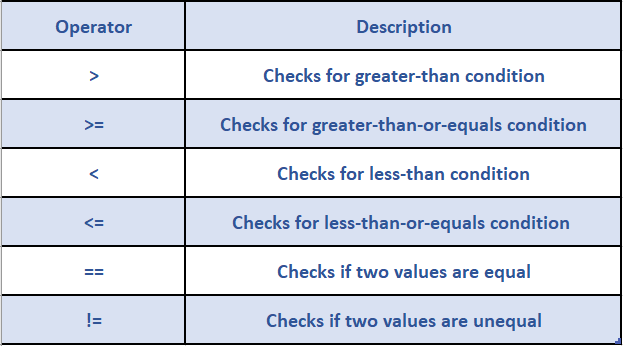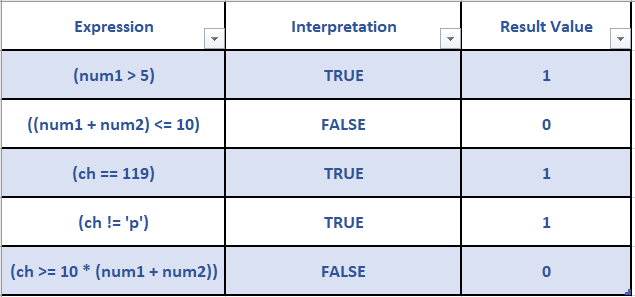In this article, we are going to understand the relational operators in C Language.

## Relational and Equality Operators:

Relational and equality operators are used to test or compare two numeric values or numeric expressions.

In C, Relational and equality operators when applied on the operands, produce an integer value which is either 0 or 1 and these are often referred to as logical values. The value 0 represents false and the value 1 represents true.

In C, there are four relational and two equality operators as given below:The format for the usage of relational and equality operators is as follows:

``operand1 operator operand2``
According to the coding conventions in C, a single space should be provided to the left and to the right of the operator.

The table given below demonstrates the use of various relational and equality operators using variables

``````int num1 = 7;
float num2 = 5.5;
char ch = 'w';``````Happy Learning 🙂